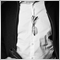# How can I start ENUM_TIMEFRAMES variables from a shift of specified minutes from their regular starting times?76

I am working with the following array of ENUM_TIMEFRAMES elements:

```ENUM_TIMEFRAMES H1andBelowTFS      = { PERIOD_M1,PERIOD_M2,PERIOD_M3,PERIOD_M4,PERIOD_M5,PERIOD_M6,PERIOD_M10,PERIOD_M12,PERIOD_M15,
PERIOD_M20,PERIOD_M30, PERIOD_H1
};```

Normally,  PERIOD_M2 starts at 00:00 and continues to 00:02, 00:04 and so on. Also PERIOD_M3 starts at 00:00 and continues to 00:03, 00:06 and so on. The other timeframes follow in a similar manner.

I am trying to figure out how to shift the starting times for the above  ENUM_TIMEFRAMES elements so that with a shift of -1 minute

PERIOD_M2 starts at 23:59 and continues to 00:01, 00:0 and so on. Also PERIOD_M3 starts at 23:59 and continues to 00:02, 00:05 and so on.

also for a shift of 1 minute

PERIOD_M2 starts at 00:01 and continues to 00:03, 00:03 and so on. Also PERIOD_M3 starts at 00:01 and continues to 00:04, 00:07 and so on.

I am using this array to compare Open, High, Low and Close of various candlesticks. Below is an example of how I am using the array to compare highs of two candles from two timeframes:

```datetime RefCandleOpenTime = NULL;
datetime RefCandleCloseTime = NULL;

RefCandleOpenTime = iTime(Symbol(),PERIOD_D1,useIndex);
RefCandleCloseTime   = datetime(ulong(iTime(Symbol(),PERIOD_D1,(useIndex-1))-1));

for(int i=ArraySize(H1andBelowTFS)-1; i>=0; i--)
{
TargetTFBar    = iBarShift(Symbol(),H1andBelowTFS[i],RefCandleOpenTime);
TargetEnd      = iBarShift(Symbol(),H1andBelowTFS[i],RefCandleCloseTime);

for(int j=TargetTFBar; j>=TargetEnd; j--)
{

candleHighA       = iHigh(Symbol(),H1andBelowTFS[i],j);
candleLowA        = iLow(Symbol(),H1andBelowTFS[i],j);
candleOpenA       = iOpen(Symbol(),H1andBelowTFS[i],j);
candleCloseA      = iClose(Symbol(),H1andBelowTFS[i],j);

//similar nested statement is used for candlesticks from another timeframe

candleHighB       = iHigh(Symbol(),H1andBelowTFS[k],l);
candleLowB        = iLow(Symbol(),H1andBelowTFS[k],l);
candleOpenB       = iOpen(Symbol(),H1andBelowTFS[k],l);
candleCloseB      = iClose(Symbol(),H1andBelowTFS[k],l);

if (candleHighA == candleHighB) {

Print("Equal High Match Found");

}

}

}

```2606

All you need is the M1 timeframe to compose any timeframe you want.

You take the open of that candle.

Then you go forward and catch the highest high and the lowest low while you move towards the time where you want to end your candle.

Then you take the close of the candle at the end.

A simple while loop will do the job for you.76

Dominik Christian Egert #:
All you need is the M1 timeframe to compose any timeframe you want.

You take the open of that candle.

Then you go forward and catch the highest high and the lowest low while you move towards the time where you want to end your candle.

Then you take the close of the candle at the end.

A simple while loop will do the job for you.

I am looking for a pattern match every few minutes. Let's say 3 minutes. I have a specified start time and I want to end at the last closed candle for each timeframe (candle index 1 for every timeframe).  How can I handle the various M1 candle residues when they are not enough to form a complete candle for one of the timeframes in the array?2606

Look at the chart and watch a 5M candle form.

If you are missing data, then you need to use what you have got. And wait for all data to be ready, so that you can close the candle.380

BluePipsOnly

I am trying to figure out how to shift the starting times for the above  ENUM_TIMEFRAMES elements so that with a shift of -1 minute

An example which may help - adjust as needed

```//+------------------------------------------------------------------+
//|                                      429580-TimeFrameShifter.mq5 |
//|                                  Copyright 2022, MetaQuotes Ltd. |
//|                                             https://www.mql5.com |
//+------------------------------------------------------------------+
#property version   "1.00"
//+------------------------------------------------------------------+
//| Script program start function                                    |
//+------------------------------------------------------------------+

//+------------------------------------------------------------------+
//|                                                                  |
//+------------------------------------------------------------------+
void OnStart()
{
ENUM_TIMEFRAMES thisTF = PERIOD_M30;
datetime        openTime = iTime(_Symbol, thisTF, 0   );
datetime        closeTime = openTime + PeriodSeconds(thisTF);

for(int min = 0; min <= 5; min++) //Minute shifter
{
PrintFormat(
"min=%i - %s OpenTime = %s Close Time = %s",
min,
EnumToString(thisTF),
TimeToString(openTime),
TimeToString(closeTime)
);
openTime    -= 60;
closeTime   -= 60;
}

}
//+------------------------------------------------------------------+
```